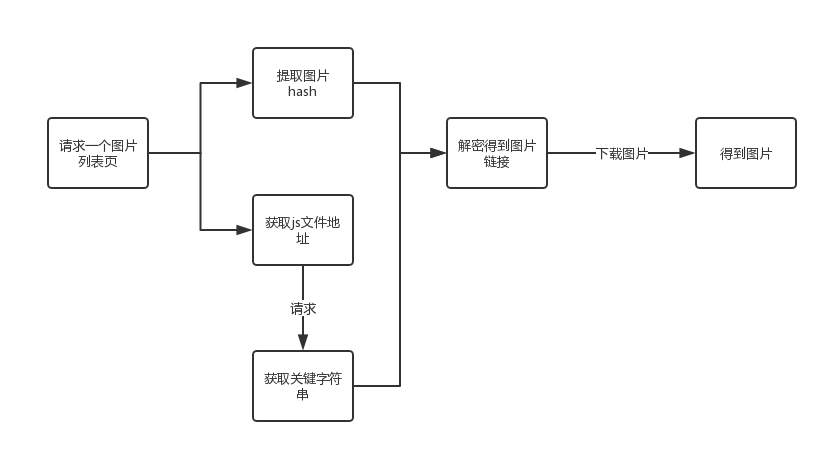## 爬虫思路分析

### 图片下载流程图### 流程图解读

2、在页面中除了提取到图片的 hash 之外，还有提取到当前页的一个关键的 js 文件的地址，这个 js 文件中包含了一个同样是用来生成图片地址的关键参数，要得到这个参数，必须去请求这个 JS 地址，当时妹子图的每个页面的 js 地址是不同的，所以需要从页面中提取。

3、得到了图片的 hash 和 js 中的关键参数之后，可以根据 js 中提供的解密方式，得到图片的链接，这个解密方式后续用 Python 代码和 js 代码的参照来说明。

4、有了图片链接，下载图片就不多说了，后续会有第二篇文章，来使用多线程+多进程的方式下载图片。

## 页面分析

### 网页源代码解读

```<p><img src="//img.jandan.net/img/blank.gif" onload="jandan_load_img(this)" /><span class="img-hash">ece8ozWUT/VGGxW1hlbITPgE0XMZ9Y/yWpCi5Rz5F/h2uSWgxwV6IQl6DAeuFiT9mH2ep3CETLlpwyD+kU0YHpsHPLnY6LMHyIQo6sTu9/UdY5k+Vjt3EQ</span></p>
```

### js 文件解读

```function jandan_load_img(b) {
var d = \$(b);
var f = d.next("span.img-hash");
var e = f.text();
f.remove();
var c = f_Qa8je29JONvWCrmeT1AJocgAtaiNWkcN(e, "agC37Is2vpAYzkFI9WVObFDN5bcFn1Px");
```

### f_ 函数的解读

```var f_Qa8je29JONvWCrmeT1AJocgAtaiNWkcN = function(m, r, d) {
var e = "DECODE";
var r = r ? r : "";
var d = d ? d : 0;
var q = 4;
r = md5(r);
var o = md5(r.substr(0, 16));
var n = md5(r.substr(16, 16));
if (q) { if (e == "DECODE") { var l = m.substr(0, q) } } else { var l = "" }
var c = o + md5(o + l);
var k;
if (e == "DECODE") {
m = m.substr(q);
k = base64_decode(m)
}
var h = new Array(256);
for (var g = 0; g < 256; g++) { h[g] = g }
var b = new Array();
for (var g = 0; g < 256; g++) { b[g] = c.charCodeAt(g % c.length) }
for (var f = g = 0; g < 256; g++) {
f = (f + h[g] + b[g]) % 256;
tmp = h[g];
h[g] = h[f];
h[f] = tmp
}
var t = "";
k = k.split("");
for (var p = f = g = 0; g < k.length; g++) {
p = (p + 1) % 256;
f = (f + h[p]) % 256;
tmp = h[p];
h[p] = h[f];
h[f] = tmp;
t += chr(ord(k[g]) ^ (h[(h[p] + h[f]) % 256]))
}
if (e == "DECODE") { if ((t.substr(0, 10) == 0 || t.substr(0, 10) - time() > 0) && t.substr(10, 16) == md5(t.substr(26) + n).substr(0, 16)) { t = t.substr(26) } else { t = "" } }
return t
};
```

### Python改写函数

```def get_imgurl(m, r='', d=0):
'''解密获取图片链接'''
e = "DECODE"
q = 4
r = _md5(r)
o = _md5(r[0:0 + 16])
n = _md5(r[16:16 + 16])
l = m[0:q]
c = o + _md5(o + l)
m = m[q:]
k = _base64_decode(m)
h = list(range(256))
b = [ord(c[g % len(c)]) for g in range(256)]

f = 0
for g in range(0, 256):
f = (f + h[g] + b[g]) % 256
tmp = h[g]
h[g] = h[f]
h[f] = tmp

t = ""
p, f = 0, 0
for g in range(0, len(k)):
p = (p + 1) % 256
f = (f + h[p]) % 256
tmp = h[p]
h[p] = h[f]
h[f] = tmp
t += chr(k[g] ^ (h[(h[p] + h[f]) % 256]))
t = t[26:]
return t
```

```var o = md5(r.substr(0, 16));
```

js 的`substr()`函数其实就是 Python 里面的切片的用法，稍微查看一下定义就能懂，不解释。

MD5 加密转化成 Python 版本如下：

```def _md5(value):
'''md5加密'''
m = hashlib.md5()
m.update(value.encode('utf-8'))
return m.hexdigest()
```

```k = base64_decode(m)
```

```binascii.Error: Incorrect padding
```

```def _base64_decode(data):
'''bash64解码，要注意原字符串长度报错问题'''
missing_padding = 4 - len(data) % 4
return base64.b64decode(data)
```

```m = 'ece8ozWUT/VGGxW1hlbITPgE0XMZ9Y/yWpCi5Rz5F/h2uSWgxwV6IQl6DAeuFiT9mH2ep3CETLlpwyD+kU0YHpsHPLnY6LMHyIQo6sTu9/UdY5k+Vjt3EQ'
r = 'HpRB2OSft5RhlSyZaXV8xYpvEAgDThcA'
print(get_imgurl(m,r))
```

```//ww3.sinaimg.cn/mw600/0073ob6Pgy1fpet9wku7dj30hs0qljuz.jpg
```

## 获取 hash 和 js 地址

### 批量获取 hash

```def get_urls(url):
'''获取一个页面的所有图片的链接'''
'user-agent': 'Mozilla/5.0 (Windows NT 6.3; Win64; x64; rv:47.0) Gecko/20100101 Firefox/47.0',
'Host': 'jandan.net'
}
js_url = 'http:' + re.findall('<script src="(//cdn.jandan.net/static/min/[\w\d]+\.\d+\.js)"></script>', html)[-1]
_r = get_r(js_url)
soup = BeautifulSoup(html, 'lxml')
tags = soup.select('.img-hash')
for tag in tags:
img_hash = tag.text
img_url = get_imgurl(img_hash,_r)
print(img_url)
```

```soup = BeautifulSoup(html, 'lxml')
tags = soup.select('.img-hash')
for tag in tags:
img_hash = tag.text
```

### 获取 js 中关键字符串

```js_url = 'http:' + re.findall('<script src="(//cdn.jandan.net/static/min/[\w\d]+\.\d+\.js)"></script>', html)[-1]
```

```def get_r(js_url):
'''获取关键字符串'''
js = requests.get(js_url).text
# 之前是使用下面注释的这个，后来煎蛋改了这个函数的名称
# _r = re.findall('c=f_[\w\d]+\(e,"(.*?)"\)', js)
_r = re.findall('c=[\w\d]+\(e,"(.*?)"\)', js)
return _r
```

## 完整代码

```# -*- coding: utf-8 -*-
import requests
from bs4 import BeautifulSoup
import hashlib
import re
import base64

def _md5(value):
'''md5加密'''
m = hashlib.md5()
m.update(value.encode('utf-8'))
return m.hexdigest()

def _base64_decode(data):
'''bash64解码，要注意原字符串长度报错问题'''
missing_padding = 4 - len(data) % 4
return base64.b64decode(data)

def get_imgurl(m, r='', d=0):
'''解密获取图片链接'''
e = "DECODE"
q = 4
r = _md5(r)
o = _md5(r[0:0 + 16])
n = _md5(r[16:16 + 16])
l = m[0:q]
c = o + _md5(o + l)
m = m[q:]
k = _base64_decode(m)
h = list(range(256))
b = [ord(c[g % len(c)]) for g in range(256)]

f = 0
for g in range(0, 256):
f = (f + h[g] + b[g]) % 256
tmp = h[g]
h[g] = h[f]
h[f] = tmp

t = ""
p, f = 0, 0
for g in range(0, len(k)):
p = (p + 1) % 256
f = (f + h[p]) % 256
tmp = h[p]
h[p] = h[f]
h[f] = tmp
t += chr(k[g] ^ (h[(h[p] + h[f]) % 256]))
t = t[26:]
return t

def get_r(js_url):
'''获取关键字符串'''
js = requests.get(js_url).text
_r = re.findall('c=[\w\d]+\(e,"(.*?)"\)', js)
return _r

def get_urls(url):
'''获取一个页面的所有图片的链接'''
'user-agent': 'Mozilla/5.0 (Windows NT 6.3; Win64; x64; rv:47.0) Gecko/20100101 Firefox/47.0',
'Host': 'jandan.net'
}
js_url = 'http:' + re.findall('<script src="(//cdn.jandan.net/static/min/[\w\d]+\.\d+\.js)"></script>', html)[-1]
_r = get_r(js_url)
soup = BeautifulSoup(html, 'lxml')
tags = soup.select('.img-hash')
for tag in tags:
img_hash = tag.text
img_url = get_imgurl(img_hash,_r)
print(img_url)

if __name__ == '__main__':
get_urls('http://jandan.net/ooxx/page-44')
```

```//ww3.sinaimg.cn/mw600/0073ob6Pgy1fpet9wku7dj30hs0qljuz.jpg
//ww3.sinaimg.cn/mw600/0073tLPGgy1fpet9mszjwj30hs0g1jsv.jpg
//ww3.sinaimg.cn/mw600/0073ob6Pgy1fpesskkgobj31jk1jkk5b.jpg
//wx3.sinaimg.cn/mw600/006XfbArly1fpesq2jn1vj30j60svaz3.jpg
//wx3.sinaimg.cn/mw600/6967abd2gy1fpenoyobrcj20u03d0b2d.jpg
//wx3.sinaimg.cn/mw600/6967abd2gy1fpenp38v9uj20u03zkhdy.jpg
```